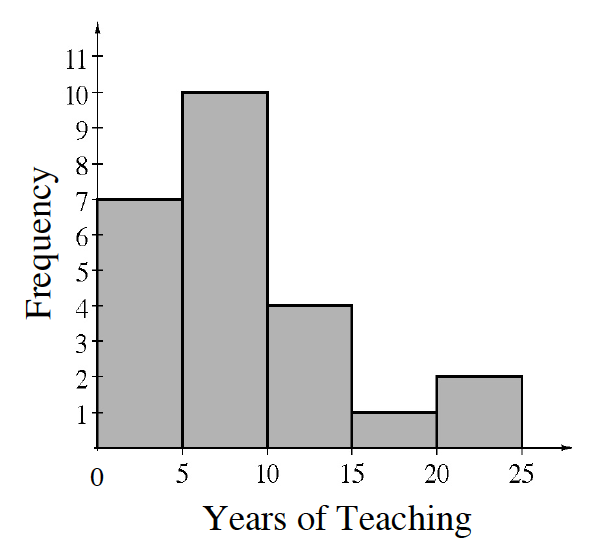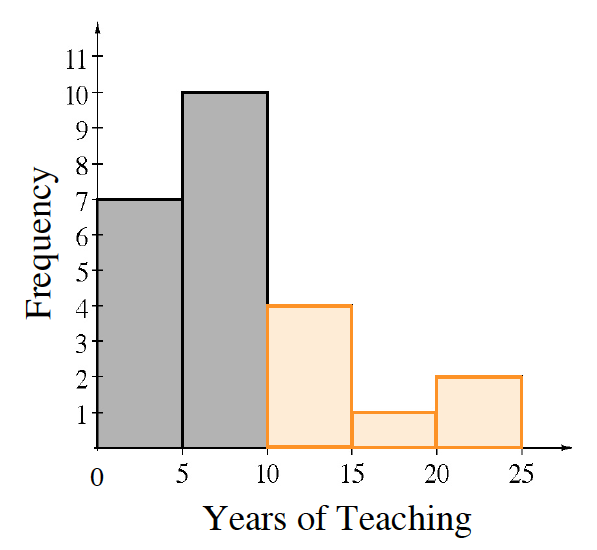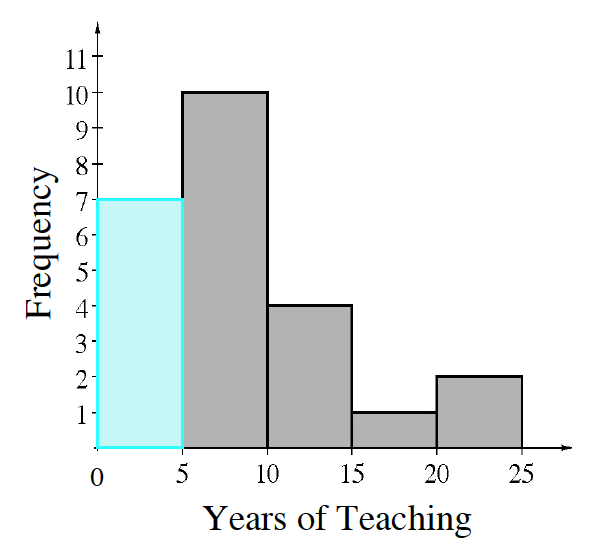### Home > CC2MN > Chapter 9 > Lesson 9.1.5 > Problem9-70

9-70.A principal made the histogram at right to analyze how many years teachers had been teaching at her school.

1. How many teachers work at her school?

Add up the frequencies represented by the bars. How many total teachers are there?

2. If the principal randomly chose one teacher to represent the school at a conference, what is the probability that the teacher would have been teaching at the school for $10$ or more years? Write the probability in two different ways.

Based on the histogram, how many teachers have been teaching for more than $10$ years? Orange shading added to the last 3 bars.

How can this fraction probability be represented as a percent?

$\frac{7}{24}$ or $\approx29\%$3. What is the probability that a teacher on the staff has been there for fewer than $5$ years?

Based on the histogram, how many teachers have been teaching for less than $5$ years? Blue shading added to the first bar, removed orange shading on last 3 bars.